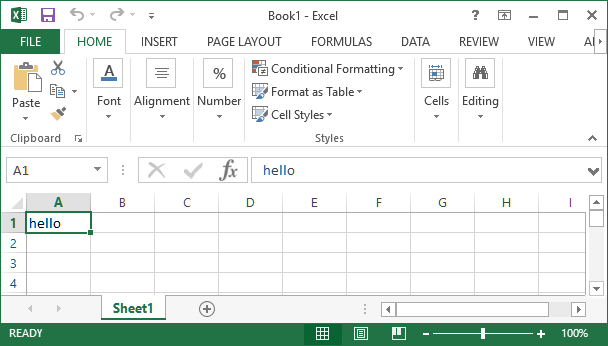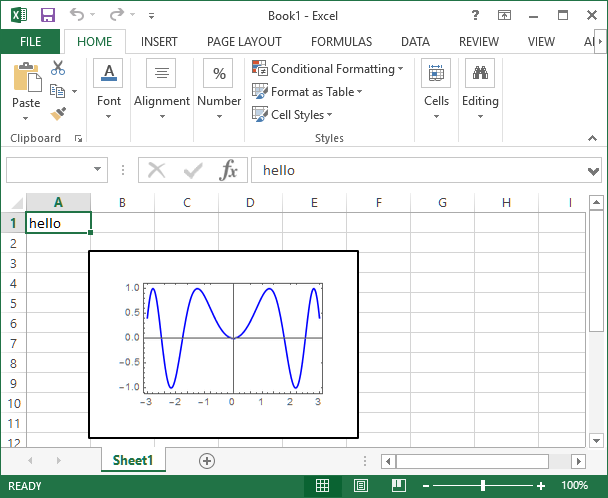# How do I get started using Excel from Mathematica?

Mathematica Link for Excel enables you to share data and calculations between Mathematica and Microsoft Excel.

Here we showcase the first few steps in using ExcelLink to connect to Excel from Mathematica. For connecting to Mathematica from Excel, see here.

Advanced information can be found in the Mathematica Link for Excel documentation.

``<< ExcelLink`` `

This loads a library of functions and symbols into Mathematica.

### Writing Text or Data to an Excel Sheet

Assign the value to the `Excel` function with the Excel cell ID as the argument. This puts the string `"hello"` in cell A1 in the active spreadsheet.

``Excel["A1"] = "hello" ``### Placing a Graphic in Excel

This creates a graphic in Mathematica and assigns it to a cell. The upper-left corner of this plot appears in cell B3.

``````g = Plot[Sin[a^2], {a, -3, 3}, PlotStyle -> Blue, Frame -> True, ImageSize -> 200];
Excel["B3"] = g ``````### Reading from an Excel sheet

Data from an Excel spreadsheet can be read into Mathematica using the `Excel` function and the range of addresses.

``Excel["A2:A6"]``

Note that numerical data is read as floating-point (real) numbers.

### Saving an Excel Sheet

Changes in an Excel spreadsheet can be saved from Mathematica using the `ExcelSave[]` function.

설명이 도움이 되었나요?

하실 말씀이 있습니까?

피드백 감사합니다.

## 지원 문의

청구서, 제품 동기화에 관한 질문에서 기술적인 질문까지 부담없이 문의하세요.전화하기

월요일 - 금요일
8am–5pm 중부 표준시

• 제품 등록 및 동기화
• 구매 전 정보 및 주문
• 설치 및 동작

## 고급 기술지원 (해당 고객을 대상으로)

월요일 - 목요일
8am–7pm 중부 표준시

금요일
8:30–10am & 11am–5pm 중부 표준시

• 우선적 기술지원
• Wolfram 전문가들의 제품 지원
• Wolfram 프로그래밍
• 고급 설치 지원